# Force of Friction examples

## Solved examples

Question 1
A block of Mass M is moving with a velocity v on straight surface.What is the shortest distance and shortest time in which the block can be stopped if μ is coefficient of friction
a. $\frac {v^2}{2 \mu g}$,$\frac {v}{\mu g}$
b. $\frac {v^2}{ \mu g}$,$\frac {v}{\mu g}$
c. $\frac {v^2}{2Mg}$,$\frac {v}{\mu g}$
d none of the above
Solution
Force of friction opposes the motion
Force of friction=μN=μmg
Therefore retardation =μmg/m=μg

From $v^2=u^2+2as$
or
$s=\frac {v^2}{2 \mu g}$

from $v=u+at$
or $t=\frac {v}{\mu g}$

Question 2
A horizontal force of F N is necessary to just hold a block stationary against a wall. The coefficient of friction between the block and the wall is μ. The weight of the block is
a. $\mu F$
b. $F(1+ \mu)$
c. $\frac {F}{\mu}$
d none of these
Solution 2
Let W be the weight
Reaction force=F
Weight downward=W
weight Upward=frictional force=μr=μF

For no movement
weight Upward=Weight downward
$W=\mu F$

Question 3.
A body is sliding down a rough inclined plane of angle of inclination θ for which coefficient of friction varies with distance y as μ(y)=Ky where K is constant.Here y is the distance moved by the body down the plane.The net force on the body is zero at y=A.Find the value of constant K
a. $\frac {tan \theta}{A}$
b. $Acot \theta$
c. $\frac {cot \theta}{A}$
d. $Atan \theta$
Solution 3
The downward force=mgsinθ
The upward force=μmgcosθ

Net force
f(y)=mgsinθ-μmgcosθ
=mg(sinθ-kycosθ)

at y=A, f(y)=0
0=sinθ-kAcosθ
or $K=\frac {tan \theta}{A}$

Question 4
A given object takes n times as much times to slide down a 45 rough incline as its takes to slide down a perfectly smooth 45 incline.The coefficient of Kinetic friction between the objects and incline is given by.
a. $\frac {1}{1-n^2}$
b. $1- \frac {1}{n^2}$
c. $\sqrt {\frac {1}{1-n^2}}$
d. $\sqrt {1- \frac {1}{n^2}}$
Solution 4

let μ be the coefficient of friction
Acceleration in smooth 45 inclined plane=$gsin \theta=\frac {g}{\sqrt {2}}$
Acceleration in friction 45 inclined plane=$gsin\theta - \mu g cos\theta=\frac {g}{\sqrt {2}}(1- \mu)$

Now $s=ut+ \frac {1}{2}at^2$
or $2s=at^2$ as u=0

For smooth plane
$2s=\frac {g}{\sqrt {2}}t^2$ ---(1)

for friction plane
$2s=\frac {g}{\sqrt {2}}(1- \mu)(nt)^2$ -(2)

so $(1- \mu)n^2=1$
or $\mu =1- \frac {1}{n^2}$

Question 5
A uniform chain of length L is lying on the horizontal surface of a table.If the coefficient of friction between the chain and the table top is μ. what is the maximum length of the chain that can hang over the edge of the table without disturbing the rest of the chain on table.?
a.L/(1+μ)
b. μL/(1+μ)
c. L/(1-μ)
d. μL/(1-μ)
Solution 5
Let m be the mass per unit length
Let L be the full length and l be the length of chain hanging
So net force downwards=mlg
Net frictional force in opposite direction=μm(L-l)g
Now mlg=μm(L-l)g
or l=μ(L-l)
or l=μL/(1+μ)

Question 6
The coefficient of static and kinetic friction between a body and the surface are .75 and .50 respectively.A force is applied to the body to make it just slide with a constant acceleration which is
a. g/4
b g/2
c. 3g/4
d g
Solution 6
Minimum force with which body will just move=μsmg
After the body start moving Frictional force becomes =μkmg
So ma=μsmg-μkmg
or a=g/4

Question 7
A block of mass M is moving with a velocity v on straight surface.What is the shortest distance and shortest time in which the block can be stopped if μ is coefficient of friction
a.v2/2μg,v/μg
b. v2/μg,v/μg
c.v2/2Mg,v/μg
d none of the above
Solution 7
Force of friction opposes the motion
Force of friction=μN=μmg
Therefore retardation =μmg/m=μg

From v2=u2+2as
or
S=v2/2μg

from v=u+at
or t=v/μg

Question 8
A horizontal force of F N is necessary to just hold a block stationary against a wall. The coefficient of friction between the block and the wall is μ. The weight of the block is
a.μF
b. F(1+μ)
c. F/μ
d none of these
Solution 8
Let W be the weight
Reaction force=F
Weight downward=W
weight Upward=frictional force=μr=μF

For no movement
weight Upward=Weight downward
W=μF

Question 9
A mass of 4 kg rests on a horizontal plane. The plane is gradually inclined until at an angle $\theta$ = 15° with the horizontal, the mass just begins to slide. What is the coefficient of static friction between the block and the surface ?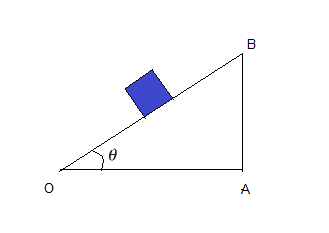Solution
First let us find the forces acting on the block
(i) the weight mg acting vertically downwards
(ii) the normal force N of the plane on the block
(iii) the static frictional force $f_s$ opposing the impending motion.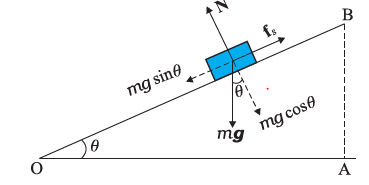In equilibrium, the resultant of these forces must be zero. Resolving the weight mg along the two directions shown, we have
$mg sin \theta = f_s$ -(1)
$mg cos \theta = N$ -(2)
As $\theta$ increases, the self-adjusting frictional force $f_s$ increases until at $\theta = \theta _{max}$, fs achieves its maximum value, $f_s max = \mu _s N$ .
Now dividing equation (1) by (2)
$tan \theta _{max} = \frac {f_s}{N} = \mu_s$
or
$\theta _{max} = tan?^{-1} \mu_s$
When $\theta$ becomes just a little more than ?max , there is a small net force on the block and it begins to slide. Note that $\theta _{max}$ depends only on $\mu _s$ and is independent of the mass of the block.
For $\theta _{max} = 15^0$,
$\mu_s = tan 15$
$= 0.27$

Question 9
What is the acceleration of the block and trolley system shown in below figure if the coefficient of kinetic friction between the trolley and the surface is 0.04? What is the tension in the string? (Take g =10 m/s-1). Neglect the mass of the string.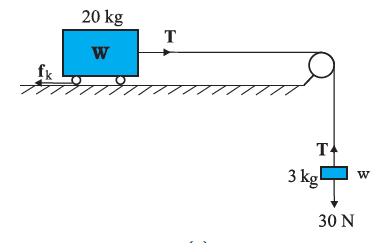Solution
Drawing free body diagram of 3 kg mass and Applying second law to motion of the block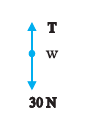$30 -? T = 3a$ -(1)
Drawing free body diagram to trollet and Applying second law to motion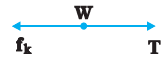$T -? f_k = 20 a$.
Now $f_k = \mu k$ N,
Here $\mu_k = 0.04$,
$N = 20 \times 10$
= 200 N.
Thus the equation for the motion of the trolley is
$T -0.04 \times 200 = 20 a$ Or $T - 8 = 20a$. -(2)
Solving equation (1) and (2),we get
a=.96 m/s2 and T=27.1 N

Question 10
A rectangular block of mass m sit on top on another similar block which in turn sits on a flat table.The maximum possible frictional force of one block on another is 2.0m N
a. Find the largest possible acceleration which can be given to lower block with out the upper block sliding off?
b. what is the coefficient of friction between the two blocks
Solution
$F_{max} = 2m= ma_{max}$
or $a_{max}=2 m/s^2$
Also
$\mu = \frac {f}{mg} = \frac {2m}{9.8m}=.20$